# Neural-Networks.ppt

5 Apr 2023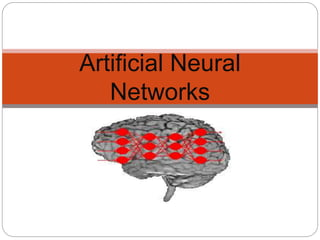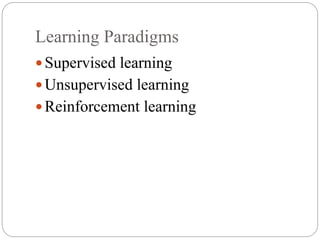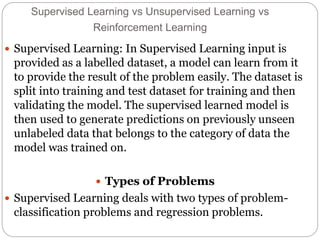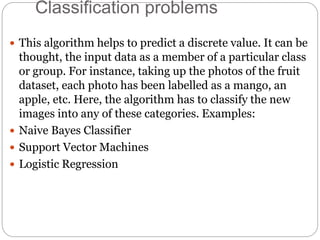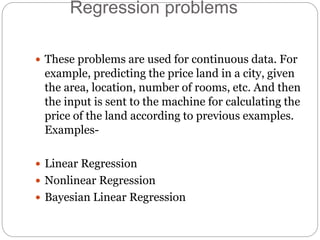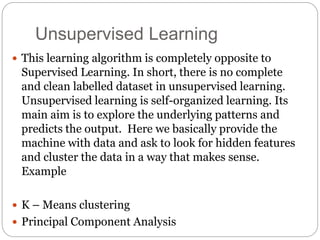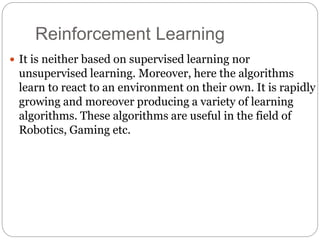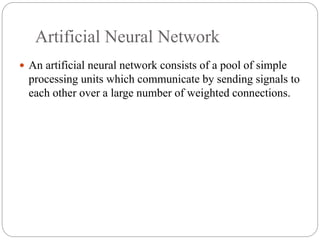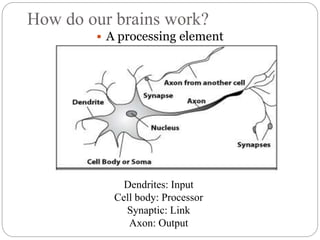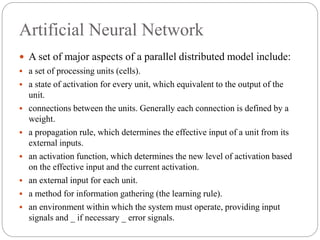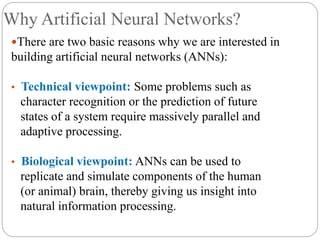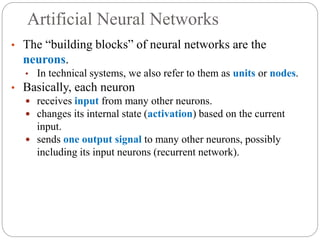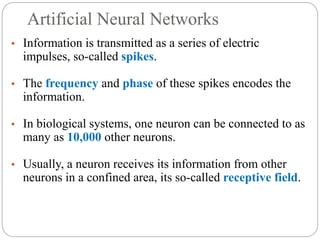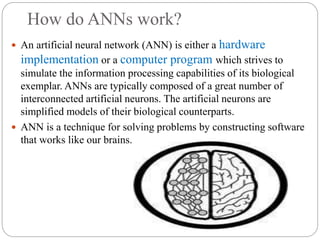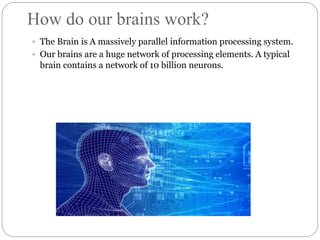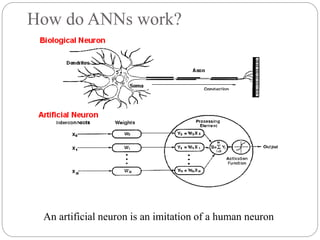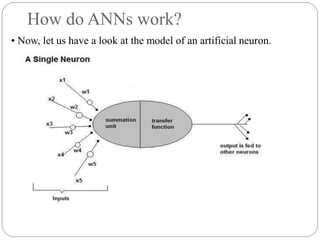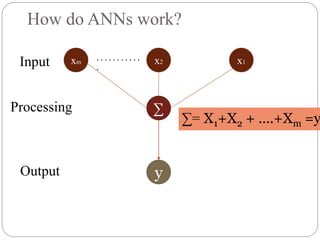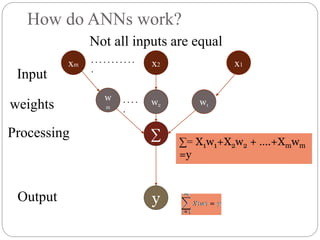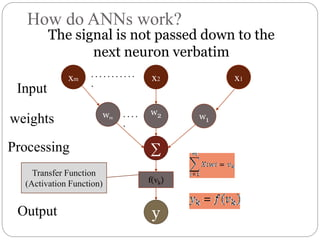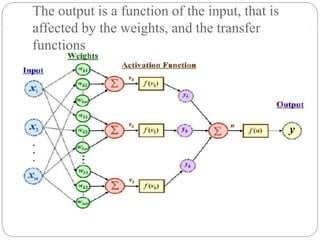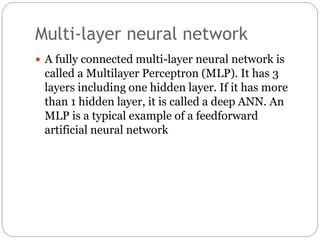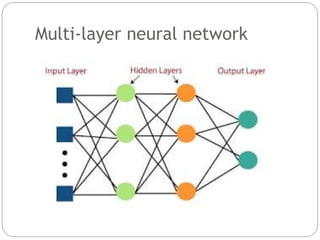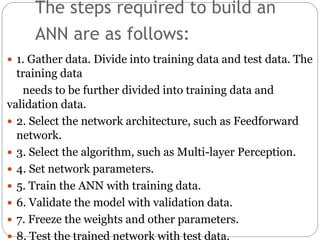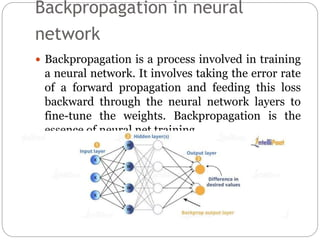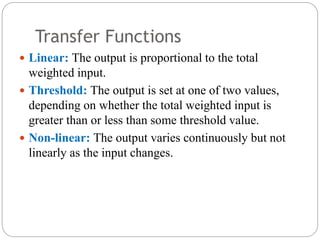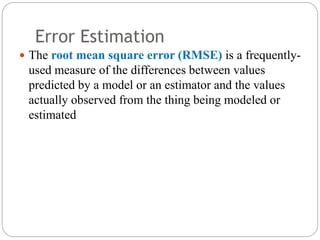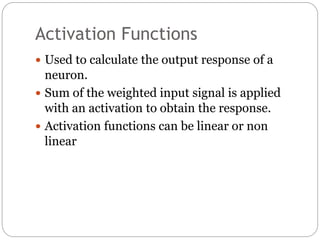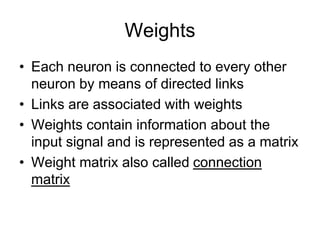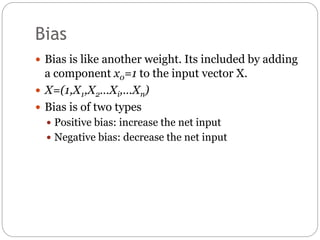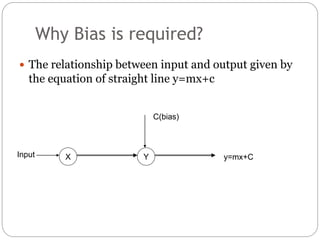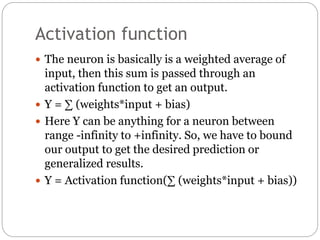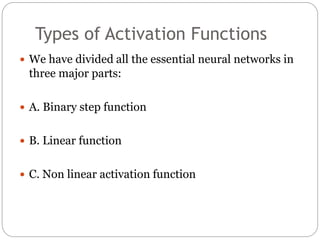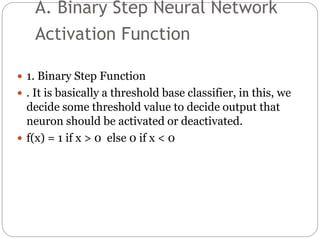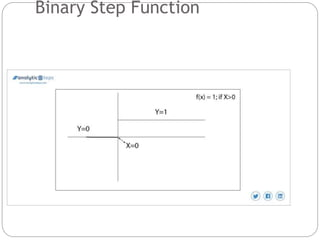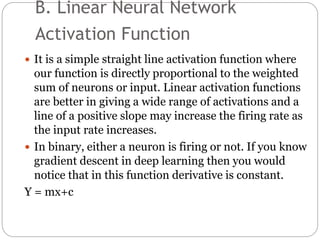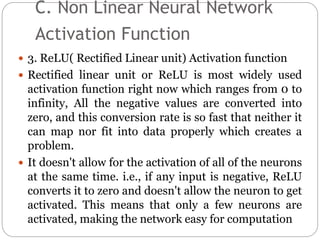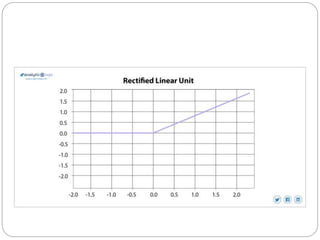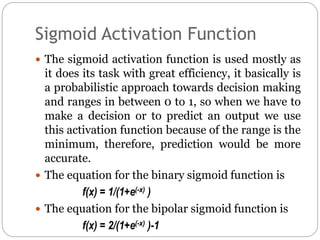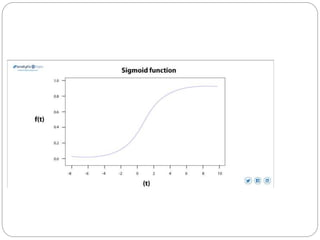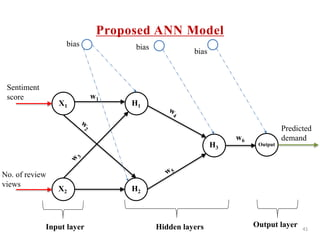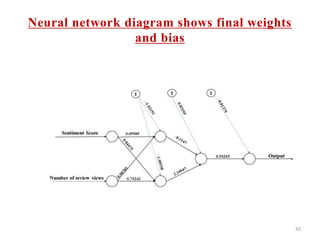1 sur 42

### Neural-Networks.ppt

• 3. Supervised Learning vs Unsupervised Learning vs Reinforcement Learning  Supervised Learning: In Supervised Learning input is provided as a labelled dataset, a model can learn from it to provide the result of the problem easily. The dataset is split into training and test dataset for training and then validating the model. The supervised learned model is then used to generate predictions on previously unseen unlabeled data that belongs to the category of data the model was trained on.  Types of Problems  Supervised Learning deals with two types of problem- classification problems and regression problems.
• 4. Classification problems  This algorithm helps to predict a discrete value. It can be thought, the input data as a member of a particular class or group. For instance, taking up the photos of the fruit dataset, each photo has been labelled as a mango, an apple, etc. Here, the algorithm has to classify the new images into any of these categories. Examples:  Naive Bayes Classifier  Support Vector Machines  Logistic Regression
• 5. Regression problems  These problems are used for continuous data. For example, predicting the price land in a city, given the area, location, number of rooms, etc. And then the input is sent to the machine for calculating the price of the land according to previous examples. Examples-  Linear Regression  Nonlinear Regression  Bayesian Linear Regression
• 6. Unsupervised Learning  This learning algorithm is completely opposite to Supervised Learning. In short, there is no complete and clean labelled dataset in unsupervised learning. Unsupervised learning is self-organized learning. Its main aim is to explore the underlying patterns and predicts the output. Here we basically provide the machine with data and ask to look for hidden features and cluster the data in a way that makes sense. Example  K – Means clustering  Principal Component Analysis
• 7. Reinforcement Learning  It is neither based on supervised learning nor unsupervised learning. Moreover, here the algorithms learn to react to an environment on their own. It is rapidly growing and moreover producing a variety of learning algorithms. These algorithms are useful in the field of Robotics, Gaming etc.
• 8. Artificial Neural Network  An artificial neural network consists of a pool of simple processing units which communicate by sending signals to each other over a large number of weighted connections.
• 9. How do our brains work?  A processing element Dendrites: Input Cell body: Processor Synaptic: Link Axon: Output
• 10. Artificial Neural Network  A set of major aspects of a parallel distributed model include:  a set of processing units (cells).  a state of activation for every unit, which equivalent to the output of the unit.  connections between the units. Generally each connection is defined by a weight.  a propagation rule, which determines the effective input of a unit from its external inputs.  an activation function, which determines the new level of activation based on the effective input and the current activation.  an external input for each unit.  a method for information gathering (the learning rule).  an environment within which the system must operate, providing input signals and _ if necessary _ error signals.
• 11. Why Artificial Neural Networks? There are two basic reasons why we are interested in building artificial neural networks (ANNs): • Technical viewpoint: Some problems such as character recognition or the prediction of future states of a system require massively parallel and adaptive processing. • Biological viewpoint: ANNs can be used to replicate and simulate components of the human (or animal) brain, thereby giving us insight into natural information processing.
• 12. Artificial Neural Networks • The “building blocks” of neural networks are the neurons. • In technical systems, we also refer to them as units or nodes. • Basically, each neuron  receives input from many other neurons.  changes its internal state (activation) based on the current input.  sends one output signal to many other neurons, possibly including its input neurons (recurrent network).
• 13. Artificial Neural Networks • Information is transmitted as a series of electric impulses, so-called spikes. • The frequency and phase of these spikes encodes the information. • In biological systems, one neuron can be connected to as many as 10,000 other neurons. • Usually, a neuron receives its information from other neurons in a confined area, its so-called receptive field.
• 14. How do ANNs work?  An artificial neural network (ANN) is either a hardware implementation or a computer program which strives to simulate the information processing capabilities of its biological exemplar. ANNs are typically composed of a great number of interconnected artificial neurons. The artificial neurons are simplified models of their biological counterparts.  ANN is a technique for solving problems by constructing software that works like our brains.
• 15. How do our brains work?  The Brain is A massively parallel information processing system.  Our brains are a huge network of processing elements. A typical brain contains a network of 10 billion neurons.
• 16. How do ANNs work? An artificial neuron is an imitation of a human neuron
• 17. How do ANNs work? • Now, let us have a look at the model of an artificial neuron.
• 18. How do ANNs work? Output x1 x2 xm ∑ y Processing Input ∑= X1+X2 + ….+Xm =y . . . . . . . . . . . .
• 19. How do ANNs work? Not all inputs are equal Output x1 x2 xm ∑ y Processing Input ∑= X1w1+X2w2 + ….+Xmwm =y w1 w2 w m weights . . . . . . . . . . . . . . . . .
• 20. How do ANNs work? The signal is not passed down to the next neuron verbatim Transfer Function (Activation Function) Output x1 x2 xm ∑ y Processing Input w1 w2 wm weights . . . . . . . . . . . . f(vk) . . . . .
• 21. The output is a function of the input, that is affected by the weights, and the transfer functions
• 22. Multi-layer neural network  A fully connected multi-layer neural network is called a Multilayer Perceptron (MLP). It has 3 layers including one hidden layer. If it has more than 1 hidden layer, it is called a deep ANN. An MLP is a typical example of a feedforward artificial neural network
• 24. The steps required to build an ANN are as follows:  1. Gather data. Divide into training data and test data. The training data needs to be further divided into training data and validation data.  2. Select the network architecture, such as Feedforward network.  3. Select the algorithm, such as Multi-layer Perception.  4. Set network parameters.  5. Train the ANN with training data.  6. Validate the model with validation data.  7. Freeze the weights and other parameters.  8. Test the trained network with test data.
• 25. Backpropagation in neural network  Backpropagation is a process involved in training a neural network. It involves taking the error rate of a forward propagation and feeding this loss backward through the neural network layers to fine-tune the weights. Backpropagation is the essence of neural net training
• 26. Transfer Functions  Linear: The output is proportional to the total weighted input.  Threshold: The output is set at one of two values, depending on whether the total weighted input is greater than or less than some threshold value.  Non‐linear: The output varies continuously but not linearly as the input changes.
• 27. Error Estimation  The root mean square error (RMSE) is a frequently- used measure of the differences between values predicted by a model or an estimator and the values actually observed from the thing being modeled or estimated
• 28. Activation Functions  Used to calculate the output response of a neuron.  Sum of the weighted input signal is applied with an activation to obtain the response.  Activation functions can be linear or non linear
• 29. Weights • Each neuron is connected to every other neuron by means of directed links • Links are associated with weights • Weights contain information about the input signal and is represented as a matrix • Weight matrix also called connection matrix
• 30. Bias  Bias is like another weight. Its included by adding a component x0=1 to the input vector X.  X=(1,X1,X2…Xi,…Xn)  Bias is of two types  Positive bias: increase the net input  Negative bias: decrease the net input
• 31. Why Bias is required?  The relationship between input and output given by the equation of straight line y=mx+c X Y Input C(bias) y=mx+C
• 32. Activation function  The neuron is basically is a weighted average of input, then this sum is passed through an activation function to get an output.  Y = ∑ (weights*input + bias)  Here Y can be anything for a neuron between range -infinity to +infinity. So, we have to bound our output to get the desired prediction or generalized results.  Y = Activation function(∑ (weights*input + bias))
• 33. Types of Activation Functions  We have divided all the essential neural networks in three major parts:  A. Binary step function  B. Linear function  C. Non linear activation function
• 34. A. Binary Step Neural Network Activation Function  1. Binary Step Function  . It is basically a threshold base classifier, in this, we decide some threshold value to decide output that neuron should be activated or deactivated.  f(x) = 1 if x > 0 else 0 if x < 0
• 36. B. Linear Neural Network Activation Function  It is a simple straight line activation function where our function is directly proportional to the weighted sum of neurons or input. Linear activation functions are better in giving a wide range of activations and a line of a positive slope may increase the firing rate as the input rate increases.  In binary, either a neuron is firing or not. If you know gradient descent in deep learning then you would notice that in this function derivative is constant. Y = mx+c
• 37. C. Non Linear Neural Network Activation Function  3. ReLU( Rectified Linear unit) Activation function  Rectified linear unit or ReLU is most widely used activation function right now which ranges from 0 to infinity, All the negative values are converted into zero, and this conversion rate is so fast that neither it can map nor fit into data properly which creates a problem.  It doesn't allow for the activation of all of the neurons at the same time. i.e., if any input is negative, ReLU converts it to zero and doesn't allow the neuron to get activated. This means that only a few neurons are activated, making the network easy for computation
• 39. Sigmoid Activation Function  The sigmoid activation function is used mostly as it does its task with great efficiency, it basically is a probabilistic approach towards decision making and ranges in between 0 to 1, so when we have to make a decision or to predict an output we use this activation function because of the range is the minimum, therefore, prediction would be more accurate.  The equation for the binary sigmoid function is f(x) = 1/(1+e(-x) )  The equation for the bipolar sigmoid function is f(x) = 2/(1+e(-x) )-1
• 41. Proposed ANN Model 41 Sentiment score Input layer Hidden layers Output layer Predicted demand No. of review views bias bias bias X1 X2 H1 Output H2 H3 w1 w6
• 42. Neural network diagram shows final weights and bias 42